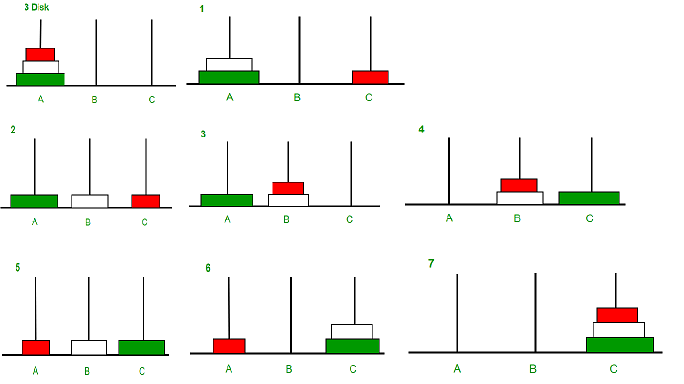# C Program for Tower of Hanoi

Tower of Hanoi is a mathematical puzzle where we have three rods and n disks. The objective of the puzzle is to move the entire stack to another rod, obeying the following simple rules:
1) Only one disk can be moved at a time.
2) Each move consists of taking the upper disk from one of the stacks and placing it on top of another stack i.e. a disk can only be moved if it is the uppermost disk on a stack.
3) No disk may be placed on top of a smaller disk.## C/C++

 `#include ` ` `  `// C recursive function to solve tower of hanoi puzzle ` `void` `towerOfHanoi(``int` `n, ``char` `from_rod, ``char` `to_rod, ``char` `aux_rod) ` `{ ` `    ``if` `(n == 1) ` `    ``{ ` `        ``printf``(``"\n Move disk 1 from rod %c to rod %c"``, from_rod, to_rod); ` `        ``return``; ` `    ``} ` `    ``towerOfHanoi(n-1, from_rod, aux_rod, to_rod); ` `    ``printf``(``"\n Move disk %d from rod %c to rod %c"``, n, from_rod, to_rod); ` `    ``towerOfHanoi(n-1, aux_rod, to_rod, from_rod); ` `} ` ` `  `int` `main() ` `{ ` `    ``int` `n = 4; ``// Number of disks ` `    ``towerOfHanoi(n, \'A\', \'C\', \'B\');  ``// A, B and C are names of rods ` `    ``return` `0; ` `} `

Output:

``` Move disk 1 from rod A to rod B
Move disk 2 from rod A to rod C
Move disk 1 from rod B to rod C
Move disk 3 from rod A to rod B
Move disk 1 from rod C to rod A
Move disk 2 from rod C to rod B
Move disk 1 from rod A to rod B
Move disk 4 from rod A to rod C
Move disk 1 from rod B to rod C
Move disk 2 from rod B to rod A
Move disk 1 from rod C to rod A
Move disk 3 from rod B to rod C
Move disk 1 from rod A to rod B
Move disk 2 from rod A to rod C
Move disk 1 from rod B to rod C
```

Please refer complete article on Program for Tower of Hanoi for more details!

My Personal Notes arrow_drop_up
Article Tags :

Be the First to upvote.

Please write to us at contribute@geeksforgeeks.org to report any issue with the above content.